# Octave-Band and Fractional Octave-Band Filters

This example shows how to design octave-band and fractional octave-band filters. Octave-band and fractional-octave-band filters are commonly used in acoustics. For example, octave filters are used to perform spectral analysis for noise control. Acousticians prefer to work with octave or fractional (often 1/3) octave filter banks because it provides a meaningful measure of the noise power in different frequency bands.

### Design of a Full Octave-Band and a 1/3-Octave-Band Filter Banks

An octave is the interval between two frequencies having a ratio of 2:1. An octave-band or fractional-octave-band filter is a bandpass filter determined by its center frequency and its order. The magnitude attenuation limits are defined in the ANSI® S1.11-2004 standard for three classes of filters: class 0, class 1 and class 2. Class 0 allow only +/-0.15 dB of ripples in the passband while class 1 filters allow +/-0.3 dB and class 2 filters allow +/-0.5 dB. Levels of stopband attenuation vary from 60 to 75 dB, depending on the class of the filter.

Design an initial full octave-band filter using the `octaveFilter` System object. This filter is the starting point for creating a filter bank.

```BW = '1 octave'; N = 6; % Filter Order F0 = 1000; % Center Frequency (Hz) Fs = 48000; % Sampling Frequency (Hz) oneOctaveFilter = octaveFilter('FilterOrder',N, ... 'CenterFrequency',F0,'Bandwidth',BW,'SampleRate',Fs)```
```oneOctaveFilter = octaveFilter with properties: FilterOrder: 6 CenterFrequency: 1000 Bandwidth: '1 octave' Oversample: false SampleRate: 48000 ```

Get all center frequencies defined by the ANSI S1.11-2004 standard in the audio range (20 Hz to 20 kHz) to design the filter bank:

```F0 = getANSICenterFrequencies(oneOctaveFilter); F0(F0<20) = []; F0(F0>20e3) = []; Nfc = length(F0); for i = 1:Nfc fullOctaveFilterBank{i} = octaveFilter('FilterOrder',N, ... 'CenterFrequency',F0(i),'Bandwidth',BW,'SampleRate',Fs); %#ok end```

Now design a 1/3-octave-band filter bank. Increase the order of each filter to 8:

```BW = '1/3 octave'; N = 8; F0 = 1000; oneThirdOctaveFilter = octaveFilter('FilterOrder',N, ... 'CenterFrequency',F0,'Bandwidth',BW,'SampleRate',Fs); F0 = getANSICenterFrequencies(oneThirdOctaveFilter); F0(F0<20) = []; F0(F0>20e3) = []; Nfc = length(F0); for i = 1:Nfc oneThirdOctaveFilterBank{i} = octaveFilter('FilterOrder',N, ... 'CenterFrequency',F0(i),'Bandwidth',BW,'SampleRate',Fs); %#ok end```

Visualize the magnitude response of one filter from each of the two filter banks. The 1/3-octave filter bank provides a finer spectral analysis but at an increased cost. It requires 30 filters versus 10 for the full octave filter bank to cover the audio range [20 20000] Hz.

`visualize(fullOctaveFilterBank{6},'class 1')`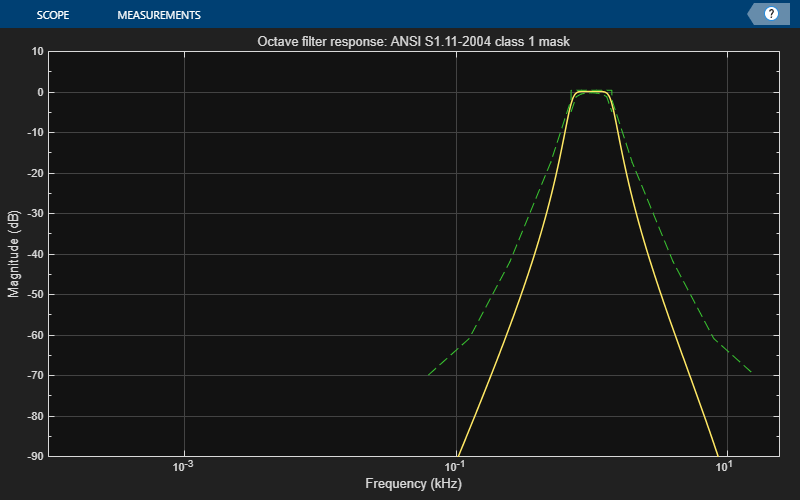`visualize(oneThirdOctaveFilterBank{6},'class 1')`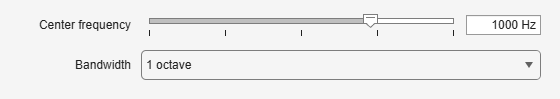The visualizer plot is synchronized to the object, so you can see the magnitude response update as you change the filter parameters. The mask around the magnitude response will be green if it complies with the ANSI S1.11-2004 standard, and red otherwise. You can also use the Audio Test Bench tool to quickly set up a test bench to use the octave filter you have designed. For example, run `audioTestBench(fullOctaveFilterBank{6})` to launch a test bench with one of the full octave filters from the filter bank.

### Spectral Analysis of White Noise

The human ear interprets loudness of sound on a scale closer to a logarithmic scale than a linear one but a DFT-based frequency analysis leads to a linear frequency scale. Compute the (DFT-based) power spectrum of a white noise signal using a spectrum analyzer:

```Nx = 100000; scope1 = dsp.SpectrumAnalyzer('SpectralAverages',50,'SampleRate',Fs, ... 'PlotAsTwoSidedSpectrum',false,'FrequencyScale','Log', ... 'YLimits',[-80 20]); tic while toc < 15 % Run for 15 seconds xw = randn(Nx,1); scope1(xw) end```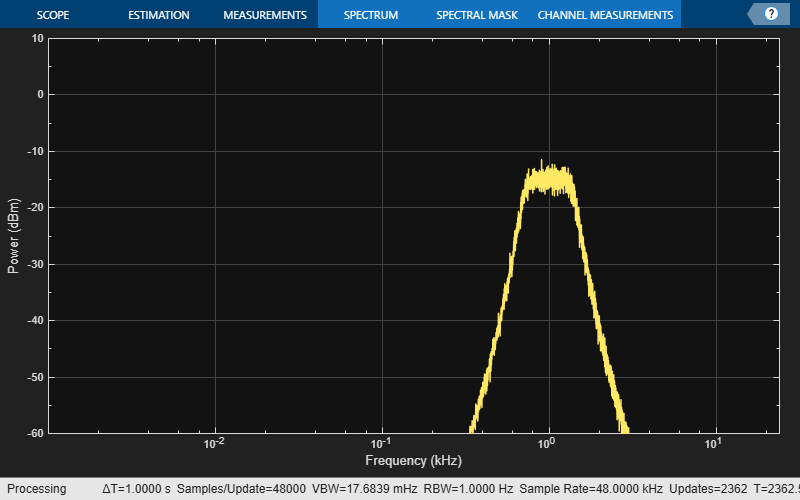Filter the white noise signal with the 1/3-octave filter bank and compute the average power at the output of each filter. While the power spectrum of a white noise signal is flat, the high frequencies are perceived louder. The 1/3-octave spectrum paints a picture that is closer to the human ear perception. It shows a spectrum where the power level rises 3 dB per octave because each band (i.e. filter) has twice the frequency range of the preceding octave band.

```scope2 = dsp.SpectrumAnalyzer('SpectralAverages',50,'SampleRate',Fs, ... 'PlotAsTwoSidedSpectrum',false,'FrequencyScale','Log', ... 'RBWSource','Property','RBW',2000); yw = zeros(Nx,Nfc); tic while toc < 15 % Run for 15 seconds xw = randn(Nx,1); for i = 1:Nfc oneThirdOctaveFilter = oneThirdOctaveFilterBank{i}; yw(:,i) = oneThirdOctaveFilter(xw); end scope2(yw) end```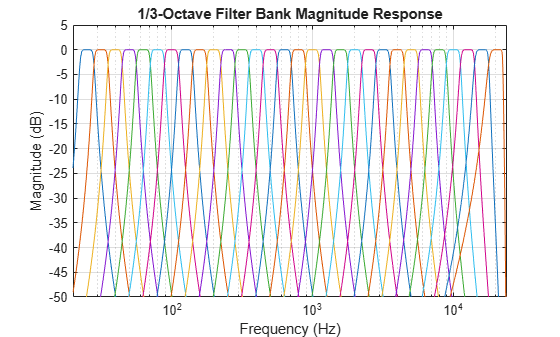### Spectral Analysis of Pink Noise

While a white noise signal has the same distribution of power for all frequencies, a pink noise signal has the same distribution of power for each octave, so the power between 0.5 Hz and 1 Hz is the same as between 5,000 Hz and 10,000 Hz.

```pinkNoise = dsp.ColoredNoise('Color','pink', ... 'SamplesPerFrame',Nx, ... 'NumChannels',1); scope3 = dsp.SpectrumAnalyzer('SpectralAverages',50,'SampleRate',Fs, ... 'PlotAsTwoSidedSpectrum',false,'FrequencyScale','Log', ... 'YLimits',[-80 20]); tic while toc < 15 % Run for 15 seconds x = pinkNoise(); scope3(x) end```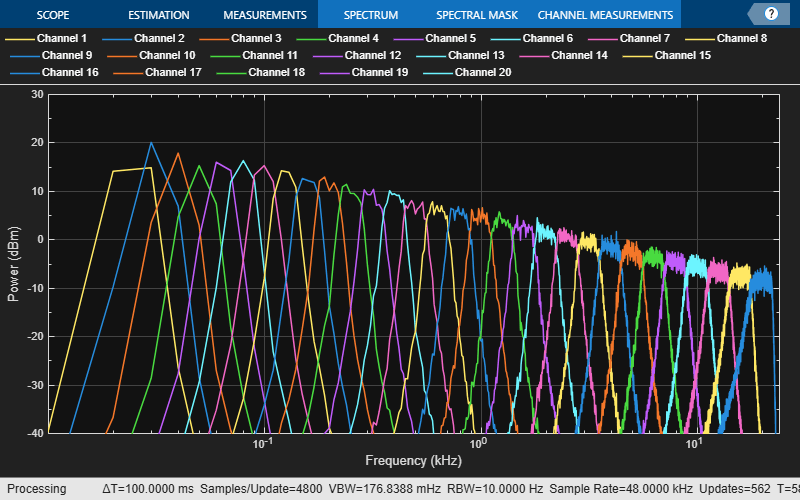Now filter the pink noise signal with the 1/3-octave filter bank and compute the average power at the output of each filter. The power of the pink noise signal declines at higher frequencies at the rate of about -3 dB per octave. However it sounds "constant" to the human hear, and the 1/3 octave-band spectrum shows flat at the output of the filter bank.

```scope4 = dsp.SpectrumAnalyzer('SpectralAverages',50,'SampleRate',Fs, ... 'PlotAsTwoSidedSpectrum',false,'FrequencyScale','Log', ... 'RBWSource','Property','RBW',2000); yp = zeros(Nx,Nfc); tic while toc < 15 % Run for 15 seconds xp = pinkNoise(); for i = 1:Nfc oneThirdOctaveFilter = oneThirdOctaveFilterBank{i}; yp(:,i) = oneThirdOctaveFilter(xp); end scope4(yp) end```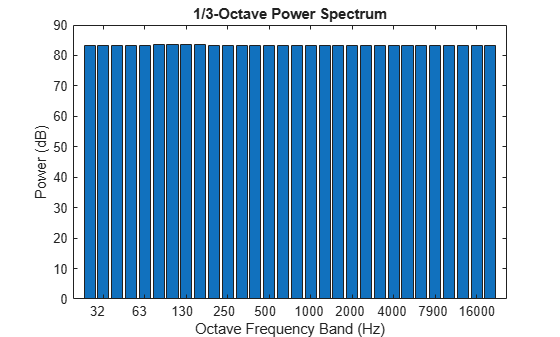##### Support평가판 신청